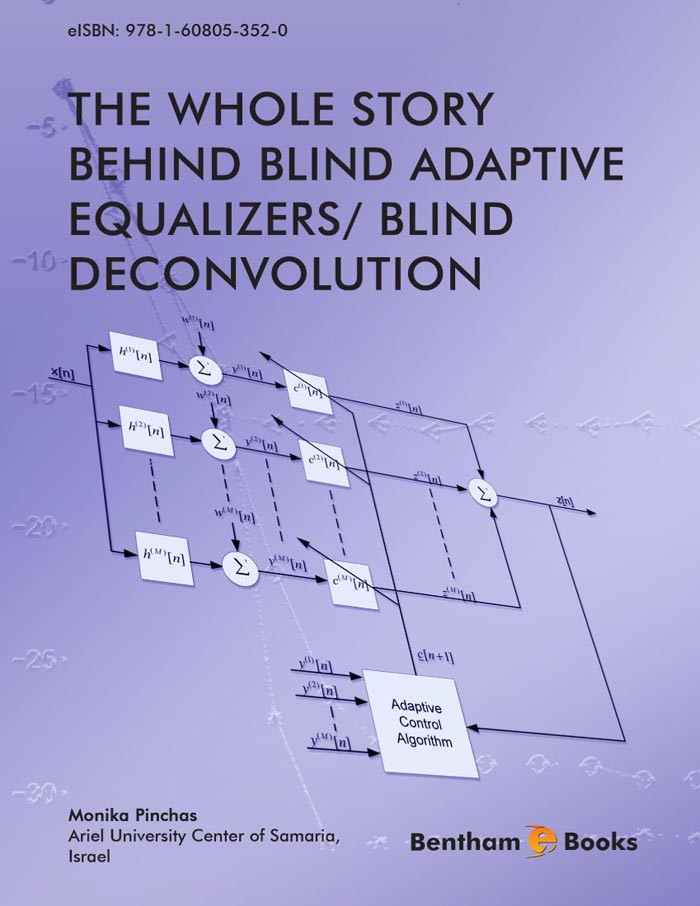### The Cost Function Approach

#### Abstract

In this chapter the cost function approach for blind deconvolution is explained and the Bayesian estimation techniques are covered. We also show the relationship between the cost function and Bayesian approach and show a derivation from the cost function approach that was recently used successfully in literature. &lt;/P&gt; &lt;P&gt; In this chapter, we prove that for the real valued and two independent quadra- ture carrier cases, no blind adaptive equalizer can be found with perfect equalization performance from the residual Intersymbol Interference (ISI) point of view inde- pendent of the source signal statistics, where the error that is fed into the adaptive mechanism which updates the equalizer&#039;s taps can be expressed as a polynomial function of the equalized output of order three and where the gain between the equalized output and source signal is equal to one. In addition, we give in this chapter the entire mathematical basis that was missing in a recently presented pa- per where a new blind adaptive equalizer was introduced and shown to have an improved equalization performance compared with Godard&#039;s algorithm. We also show that the above mentioned blind adaptive equalizer was obtained by using a derivation from the cost function approach.

Total Pages: 23-42 (20)